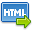Volume No. :   9

Issue No. :  1

Year :  2017

ISSN Print :  0975-4393

ISSN Online :  2349-2988Registration

## Applications of Mathematics in Chemistry –II

 Address: Anil Kumar Sharma Department of Chemistry, Govt. Degree College, Bhoranj (Tarkwari), Distt. Hamirpur, Himachal Pradesh, INDIA *Corresponding Author
DOI No: 10.5958/2349-2988.2017.00017.1

ABSTRACT:
Mathematics is widely used in chemistry as well as all other sciences. Mathematical calculations are absolutely necessary to explore important concepts in chemistry. Without some basic mathematical skills, these calculations, and therefore chemistry itself will be extremely difficult. In the present paper mathematical equations in excess volumes and thermodynamic transfer properties are discussed Experimental excess volume results are analyzed in the light of the Prigogine Flory Patterson theory. Theory divide excess volume value into three contributions. The interactional contribution, V ? curvature contribution and P* contribution. All these three contributions are calculated mathematically. In thermodynamic Studies of electrolytes in aqueous solutions from electromotive force measurements are discussed. From electromotive force values of a cell E_m^? and activity coefficient ?± are calculated by mathematical equations. The values of ?± give idea about solute solvent interactions. Then Gibbs free energy of transfer, ??G?_t^?, for electrolyte from water to aqueous solution are calculated from mathematical equations and results are interpreted in terms of spontaneous process for transfer of electrolyte and comparison of Basic character of water and aqueous solutions. Further By mathematical calculations ??G?_t^? is divided into two parts ??G?_(t,el)^? and ??G?_(t,ch)^?.
KEYWORDS:
Applications of Mathematics in Chemistry –II.
Cite:
Anil Kumar Sharma. Applications of Mathematics in Chemistry –II. Research J. Science and Tech. 2017; 9(1):115-117.[View HTML][View PDF]

Visitor's No. :   217285